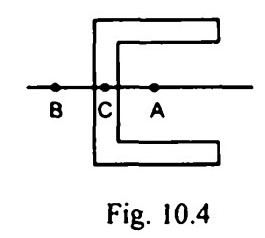Search

# Strength of Materials(61-80)

Updated: Apr 9, 2020

61.A beam of square cross-section with side 100 mm is placed with one diagonal

vertical. If the shear force acting on the section is 10 kN, the maximum shear

stress is 1.125 N/mm2

62. A prismatic bar when subjected to pure bending assumes the shape of

arc of a circle

63. A beam of triangular cross section is placed with its base horizontal. The

maximum shear stress intensity in the section will be above the neutral axis

64. A beam of I-section 600 mm deep and 200 mm wide has flanges 25 mm thick and web 20 mm thick. If the shear stress in the web at the junction of flange and web is q, then the shear stress in the flange at the junction is 0.1q

65. A beam of uniform strength has at every cross-section same bending stress

66.The shear centre in the channel section shown in Fig. 10.4 will be at B

67. tor no torsion, the plane of bending v should pass through shear centre of section

68. Two beams, one of circular cross-section and other of square cross-section, have equal areas of cross-section. If subjected to bending square section is more economical

69. The portion, which should be removed from top and bottom of a circular cross

section of diameter d in order to obtain maximum section modulus, is 0.011d

70. A beam of overall length / rests on two simple supports with equal overhangs on both sides. Two equal loads act at the free ends. If the deflection at the centre of the beam is the same as at either end, then the length of either overhang is

0.152 l

71. A beam ABC rests on simple supports at A and B with BC as an overhang. D is

centre of span AB. If in the first case a concentrated load P acts at C while in the

second case load P acts at D, then the deflection at D in the first case will be

equal to the deflection at C in the second case

72. Slope at the end of a simply supported beam of span 1 with uniformly distributed load w/unit length over the entire span is given by73.If the deflection at the free end of a uniformly loaded cantilever beam is

15mm and the slope of the deflection curve at the free end is 0.02 radian, then

the length of the beam is 1 m

74.A simply supported beam ABC of length 1 carries a concentrated load P at an

intermediate point B. If the slope at A is 0.75 times the slope at C, then the length

of portion AB is equal to 5/7 l

75.If the deflection at the free end of a uniformly loaded cantilever beam of

length 1 m is equal to 7.5 mm, then the slope at the free end is 0.02 radian

76. A simply supported beam of circular cross-section with diameter d and length 1 carries a concentrated load W at the centre of the beam. The strength of the beam is proportional to

77. A cantilever beam carries a uniformly distributed load from fixed end to the

centre of the beam in the first case and a uniformly distributed load of same intensity from centre of the beam to the free end in the second case. The ratio of

deflections in the two cases is 7/41

78. If the length of a simply supported beam carrying a concentrated load at the centre is doubled, the defection at the centre will become eight times

79. A simply supported beam with rectangular cross-section is subjected to a central concentrated load. If the width and depth of the beam are doubled, then the deflection at the centre of the beam will be reduced to 6.25%

80.A laminated spring is given an initial curvature because spring becomes flat when it is subjected to design load